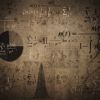# Mathematics

## Famous quotes and proverbs

|
|
|
|
|
Page: 1 2
 I've always considered myself more of a mathematician than a psychologist. » Alan Greenspan  (1926-?) American Economist We can only see a short distance ahead, but we can see plenty there that needs to be done. » Alan Turing  (1912-1954) English Mathematician Mathematical reasoning may be regarded rather schematically as the exercise of a combination of two facilities, which we may call intuition and ingenuity. » Alan Turing  (1912-1954) English Mathematician The shortest distance between two points is a straight line. » Archimedes  (287-212) Greek Mathematician I mean the word proof not in the sense of the lawyers, who set two half proofs equal to a whole one, but in the sense of a mathematician, where half proof = 0, and it is demanded for proof that every doubt becomes impossible. » Carl Friedrich Gauss  (1777-1855) German Mathematician The problem of distinguishing prime numbers from composite numbers and of resolving the latter into their prime factors is known to be one of the most important and useful in arithmetic. » Carl Friedrich Gauss  (1777-1855) German Mathematician There is no philosophy which is not founded upon knowledge of the phenomena, but to get any profit from this knowledge it is absolutely necessary to be a mathematician. » Daniel Bernoulli  (1700-1782) Dutch Mathematician It would be better for the true physics if there were no mathematicians on earth. » Daniel Bernoulli  (1700-1782) Dutch Mathematician Hypotheses are what we lack the least. » Henri Poincaré  (1854-1912) French Mathematician Point set topology is a disease from which the human race will soon recover. » Henri Poincaré  (1854-1912) French Mathematician Mathematicians do not study objects, but relations between objects. » Henri Poincaré  (1854-1912) French Mathematician One would have to have completely forgotten the history of science so as to not remember that the desire to know nature has had the most constant and the happiest influence on the development of mathematics. » Henri Poincaré  (1854-1912) French Mathematician
 Science is a differential equation. Religion is a boundary condition. » Alan Turing  (1912-1954) English Mathematician Mathematicians stand on each other's shoulders. » Carl Friedrich Gauss  (1777-1855) German Mathematician It may be true, that men, who are mere mathematicians, have certain specific shortcomings, but that is not the fault of mathematics, for it is equally true of every other exclusive occupation. » Carl Friedrich Gauss  (1777-1855) German Mathematician It is the harmony of the diverse parts, their symmetry, their happy balance; in a word it is all that introduces order, all that gives unity, that permits us to see clearly and to comprehend at once both the ensemble and the details. » Henri Poincaré  (1854-1912) French Mathematician Geometry is not true, it is advantageous. » Henri Poincaré  (1854-1912) French Mathematician Mathematics is the art of giving the same name to different things. » Henri Poincaré  (1854-1912) French Mathematician
Page: 1 2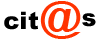Frases célebres y piropos de amor en tu email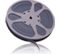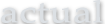30 November 2023

# There is nothing in the world like a persuasive speech to fuddle the mental apparatus.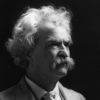## Mark Twain

### Speakers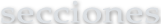###Cartas de amor

Declaraciones de amor y cartas para dedicar

###Dedicatorias

Frases originales para dedicar en cada ocasión

###Literatura

Recorrido por las etapas de la literatura española

###Relatos breves

Kafka, Hemingway, Agatha Christie, Sherlock Holmes

###Cuentos

Grandes clÃ¡sicos de Andersen, Grimm, Perrault, ...

###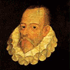Biblioteca

Ediciones digitales de grandes clásicos

###Ebooks gratis

Clásicos literarios en formato ebook para descargar

###Multicultural

Web dedicada a los escritores multiculturales